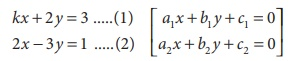Home | | Maths 9th std | Consistency and Inconsistency of Linear Equations in Two Variables

# Consistency and Inconsistency of Linear Equations in Two Variables

Consistency and Inconsistency of Linear Equations in Two Variables

Consistency and Inconsistency of Linear Equations in Two Variables

Consider linear equations in two variables say

a1 x + b1 y + c1 = 0 ...(1)

a2 x + b2 y + c2 = 0 ...(2)

where a 1 , a2 , b1 , b2 , c1 and c2 are real numbers.

Then the system has :Example 3.54

Check whether the following system of equation is consistent or inconsistent and say how many solutions we can have if it is consistent.

(i) 2x – 4y = 7; x – 3y = –2

(ii) 4x + y = 3 ; 8x + 2y = 6

(iii) 4x +7 = 2 y ; 2x + 9 = y

SolutionExample 3.55

Check the value of k for which the given system of equations kx + 2y = 3; 2x 3y = 1 has a unique solution.

### Solution

Given linear equations are

kx + 2 y = 3 .....(1) [a1 x + b1 y + c1 = 0]

2x 3y = 1 .....(2)   [a x + b y + c = 0 ]Here a1 = k, b1 = 2, a2 = 2, b2 = −3 ;

For unique solution we take a1/a2b1/b2 ; therefore k/2 ≠ 2/-3 ; k ≠ 4/-3 , that is k ≠ − 4/3 .

### Example 3.56

Find the value of k, for the following system of equation has infinitely many solutions. 2x 3y = 7; (k + 2)x (2k + 1)y = 3(2k 1)

### Solution

Given two linear equations are

2x − 3y = 7  [a1 x + b1 y + c1 = 0 ]

(k + 2)x − (2k + 1)y = 3(2k − 1) [a2x + b2 y + c2 = 0 ]Here a1 = 2, b1 = −3, a2 = (k + 2), b2 = −(2k + 1), c1 = 7, c2 = 3(2k −1)

For infinite number of solution we consider### Example 3.57

Find the value of k for which the system of linear equations 8x + 5y = 9; kx + 10 y = 15 has no solution.

Solution Given linear equations are

8x + 5y = 9  [ a1 x + b1 y + c1 = 0]

kx + 10 y = 15 [a2 x + b2 y + c2 = 0]Here a1 = 8, b1 = 5, c1 = 9, a2 = k , b2 = 10, c2 = 15

For no solution, we know thata1/a2 = b1/b2 ≠ c1/c2 and so, 8/k = 5/10 ≠ 9/15

80 = 5k

k= 16

### Activity – 3

1. Find the value of k for the given system of linear equations satisfying the condition below:

(i) 2x + ky = 1; 3x 5y = 7 has a unique solution

(ii) kx + 3y = 3; 12x + ky = 6 has no solution

(iii) (k 3)x + 3y = k; kx + ky = 12 has infinite number of solution

Solution:2. Find the value of a and b for which the given system of linear equation has infinite number of solutions 3x (a + 1)y = 2b 1, 5x + (1 2a)y = 3b

Solution:### Activity – 4

For the given linear equations, find another linear equation satisfying each of the given conditionSolution:Tags : Example Solved Problems | Algebra | Maths , 9th Maths : UNIT 3 : Algebra
Study Material, Lecturing Notes, Assignment, Reference, Wiki description explanation, brief detail
9th Maths : UNIT 3 : Algebra : Consistency and Inconsistency of Linear Equations in Two Variables | Example Solved Problems | Algebra | Maths